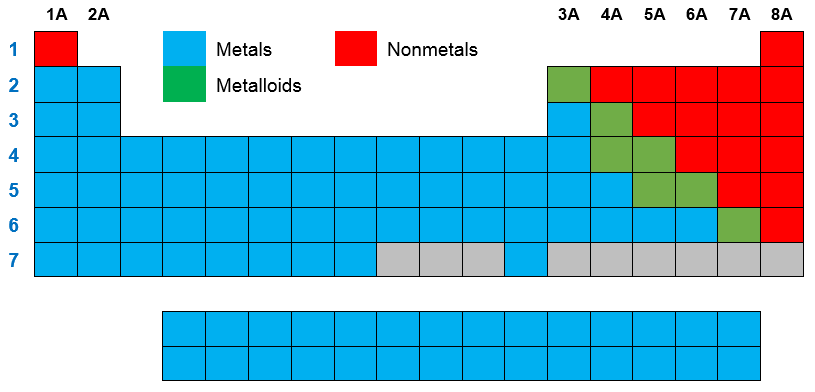Chemistry Practice Problems Periodic Table Practice Problems Solution: Which of the following does NOT describe a metal?A...

🤓 Based on our data, we think this question is relevant for Professor Barnes' class at UWF.

# Solution: Which of the following does NOT describe a metal?A) Found on the left side of the periodic table.B) Good conductor of heat.C) Form ionic compounds with nonmetals.D) Good conductor of electricity.E) Tend to gain electrons.

###### Problem

Which of the following does NOT describe a metal?

A) Found on the left side of the periodic table.

B) Good conductor of heat.

C) Form ionic compounds with nonmetals.

D) Good conductor of electricity.

E) Tend to gain electrons.

###### Solution

We’re being asked to determine which of the given statements does not describe a metal.

Let’s analyze each given statement:

A) Found on the left side of the periodic table.

If you take a look at the illustration of the periodic table below:View Complete Written Solution

Periodic Table

Periodic Table

#### Q. Which ions should be formed by magnesium, nitrogen, and chlorine, respectively? (A) Mg2+, N3-, Cl- (B) Mn7+, N3-, C4+ (C) Mg2+, N5+, Cl- (D) Mg+, ...

Solved • Wed Jul 12 2017 03:51:08 GMT-0400 (EDT)

Periodic Table

#### Q. Which of the following elements is NOT correctly matched with its symbol? (A) sodium is Na (B) phosphorus is P (C) chlorine is Cl (D) carbon is C ...

Solved • Wed Jul 12 2017 03:13:29 GMT-0400 (EDT)

Periodic Table

#### Q. What type of ions would be formed by potassium, aluminum and oxygen atoms, respectively? (A) P+, Al3+, O2- (B) K+, Al3+, O2- (C) K+, Al+, O6+ (D) ...

Solved • Tue Jul 11 2017 22:17:45 GMT-0400 (EDT)

Periodic Table

#### Q. All the following elements exist as diatomic gases at room temperature and atmospheric pressure except H Cl N Ar O

Solved • Mon Jul 10 2017 08:56:20 GMT-0400 (EDT)